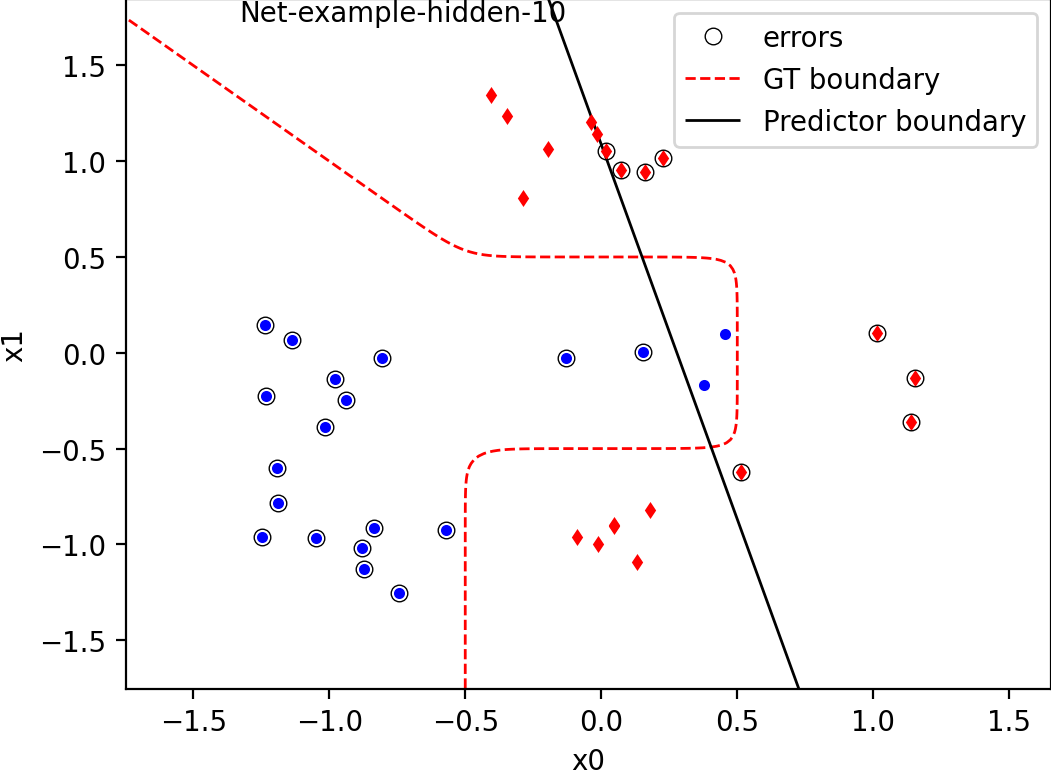Quick links: Schedule | Forum | BRUTE | Lectures | Labs

# Lab 1: Preparations, Double Descent

This lab has an introduction part, explaining how the study process is organized and what tools you need to work with; and a light homework part.

Preparations:

• How To: – software, python IDEs

### Double Descent (5p)

In this and the next lab we will work with a simulated data for binary classification of 2D features drawn from a known Gaussian mixture model. Such toy data allows to visualize all the classifiers and is already sufficient to observe several interesting phenomena.

#### Templates

Use the provided template. The package contains:

• tools for working with the ground truth model: loading, generating, plotting the decision boundary of a classifier versus the optimal classifier implemented as a class G2Model.
• example usage code in “main”

#### Classification Problem

Let $x$ denote observations in $\mathbb{R}^2$ and $y\in\{-1,1\}$ denote the class label. In this lab we have chosen an artificial ground truth model $p(x,y)$, accessible to you through functions of the class G2Model. You can generate a training set and a test set yourself using the function generate_sample, which samples from this ground truth model. You can also access the optimal Bayesian classification according to the true model (function classify) and compare it to the classification by you neural network.

The next plot show an example of 40 data points, the GT decision boundary and a linear classifier boundary, drawn with plot_boundary(train_data, net):The goal of this lab is to observe a phenomenon which is somewhat counterintuitive and contradicting to the classical learning theory. The phenomenon is called double descent and is described in the paper: Belkin et al. 2018. Reconciling modern machine-learning practice and the classical bias–variance trade-off. We will replicate the experiment in a simple scenario described in Appendix C.2. of the paper. The classical learning theory (in particular structural risk minimization) suggests that when increasing the model capacity, we will be eventually overfitting to the data and generalize poorly. Therefore there should be a tradeoff between the training error and the model complexity. This experiment gives one example when it is not exactly so…

We will consider a neural network with one hidden layer: \begin{aligned} & s = W_2({\rm ReLU}(W_1 x + b_1)) + b_2\\ & p(y=1|x) = {\rm sigmoid}(s) \end{aligned} The first layer weights and biases will not be learned but just randomly initialized. Use the following code snippet for their initialization:

W1 = (np.random.rand(hidden_size, input_size) * 2 - 1)
W1 /= np.linalg.norm(W1, axis=1).reshape(hidden_size, 1)
b1 = (np.random.rand(hidden_size) * 2 - 1) * 2
This ensures that hidden units can partition the input domain into random half-spaces that can pass more or less anywhere around the data. In this way the first layer, provides a randomized feature lifting of the initial data by performing a random linear projection and computing a simple non-linearity given by ${\rm ReLU}$. We then want to train the second layer, i.e. the affine transform defined by $W_2, b_2$.

In this experiment, we will use the MSE loss as the training loss function, i.e. $$\mathcal{L}(\theta) = \frac{1}{n}\sum_i \|s_i - y_i\|^2,$$ where $s_i$ is the network score for the data point $x_i$; $y_i$ is the class label encoded as $\{-1,1\}$ and $\theta = (W_2, b_2)$. In our case $W_2$ is a matrix of size $1 \times {\rm hidden\_size}$ and thus $\theta$ is a vector of size ${\rm hidden\_size} + 1$. Optimizing this loss in the parameters of the last layer only is equivalent to just the linear regression. Thus, we can avoid time consuming gradient descent optimization by using the closed form solution for linear regression. Recall that the solution can be computed as $$\theta = (X^{\sf T} X + \lambda I)^{-1} X^{\sf T} y,$$ where $X$ is the data matrix and $\lambda=10^{-5}$ is introduced for numerical stability. In our case the rows of the data matrix $X$ are formed by the random first-layer features ${\rm ReLU}(W_1 x_i + b_1)$, augmented with $1$ (so that the score of the model is given by $X \theta$).

##### Step 1 (2p)

Use the training set size of 200 points and $\rm{hidden\_size}=10$. Find the optimal setting of parameters $W_2, b_2$ by linear regression. Plot the classifier decision boundary. Report its training and test error.

##### Step 2 (2p)

Use the training set size of 40 points. Vary the number of hidden units from 1 to 100 (in steps of 2 to 10) and for each $\rm{hidden\_size}$ solve the linear regression problem as discussed above, compute its train and test MSE losses and error rates. Plot the losses and error rates versus $\rm{hidden\_size}$. The result will depend on the random initialization of the first layer. Repeat the experiment for $T=100$ trials and average the loss and error graphs over all trials. Report two figures:

1. train and test average MSE loss vs $\rm{hidden\_size}$ in one plot.
2. train and test accuracy vs $\rm{hidden\_size}$ in one plot.
• Do you observe the classical U-shape for hidden size in between 1 and 40 (our training data size)?
• Do you observe something interesting for $\rm{hidden\_size}$ above 40?
##### Step 3 (1p)

Repeat the above experiment when the training labels are noisy. More specifically, let each training label be flipped with probability $0.05$ (respectively, retain its correct value with probability $0.95$). Does the peak in the test performance become more prominent?

##### Discussion

We have investigated a rather special example and linear regression. Does it apply to deep networks? Read about Deep Double Descent on open.ai.

##### Submit

Submit the report to BRUTE:

• Saved jupyter notebook with results or PDF report.
• Source code.
• It is ok if the report contains only what we asked for. But you can also explore more, write your thoughts and get individual feedback.